Search QuantityWare

# BCP Configuration FAQs

Answers to common questions regarding configuring Bulk Calculations – Petroleum (BCP) on your systems

### How can I extend the density and temperature range for my product?

I have a product which has a base density beyond the supported measurement standard range, is it possible to extend the calculation range?

If the algorithm defined in the measurement standards allows so, you simply extend the range for densities, pressures and temperatures by selecting the “Extend range of standard implementation” indicator in the conversion group details screen:Read Annex D: Standard Range Limit Extensions in the BCP Supported Standards Manual for additional information.

Important: If you decide to set the “extend range of standard implementation” indicator,

### We have extended the ranges of our ASTM D1250-04 conversion group and receive a short dump - how can we prevent such a dump?

Answer: You need to define technical ranges for your conversion group observed density value.

Example: You have extended the ranges for conversion group Q210: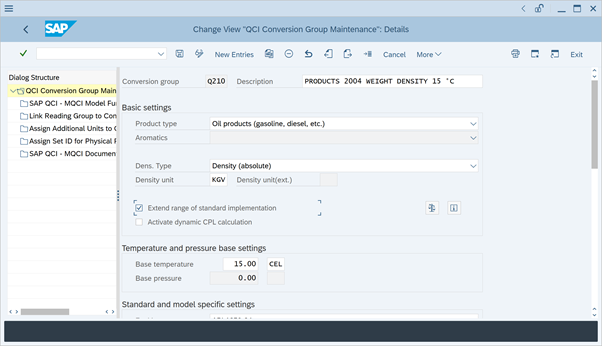You perform a trial calculation with a density of 0 and a UoM (here KGV) and hit “Return”: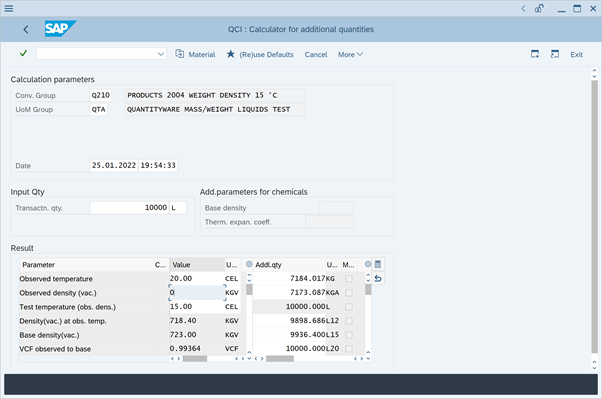An ABAP short dump happens: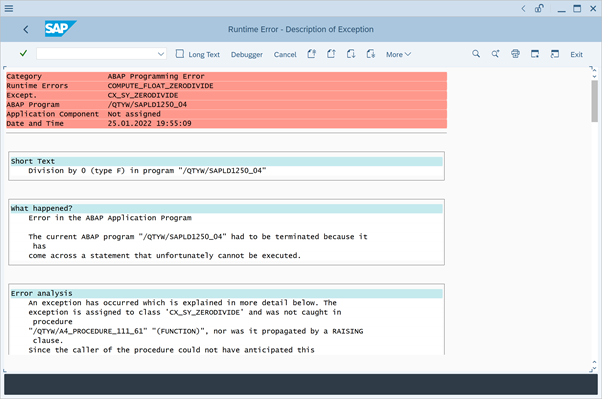The zero density value leads to a division by zero error. In order to prevent this, you must define a technical range check for the observed density:

In the Petroleum Measurement Cockpit (PMC), you navigate to the range group customizing settings: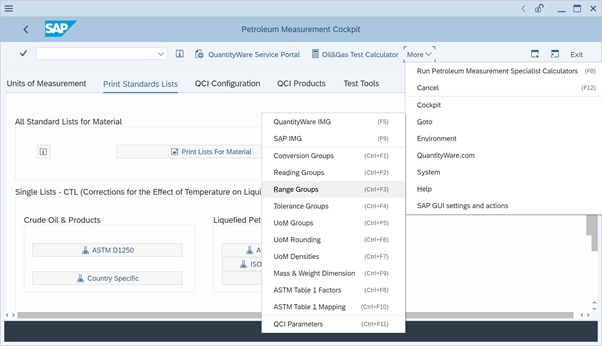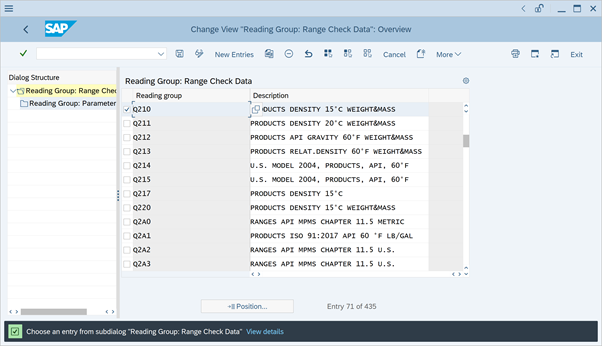4 input parameters are defined in the reading group –

### For our crude oil conversion group, the S&W % value increases the product mass and weight instead of decreasing it. How can this be?

Example:

Enter a transaction quantity of 10 000 m³ (SAP UoM M3):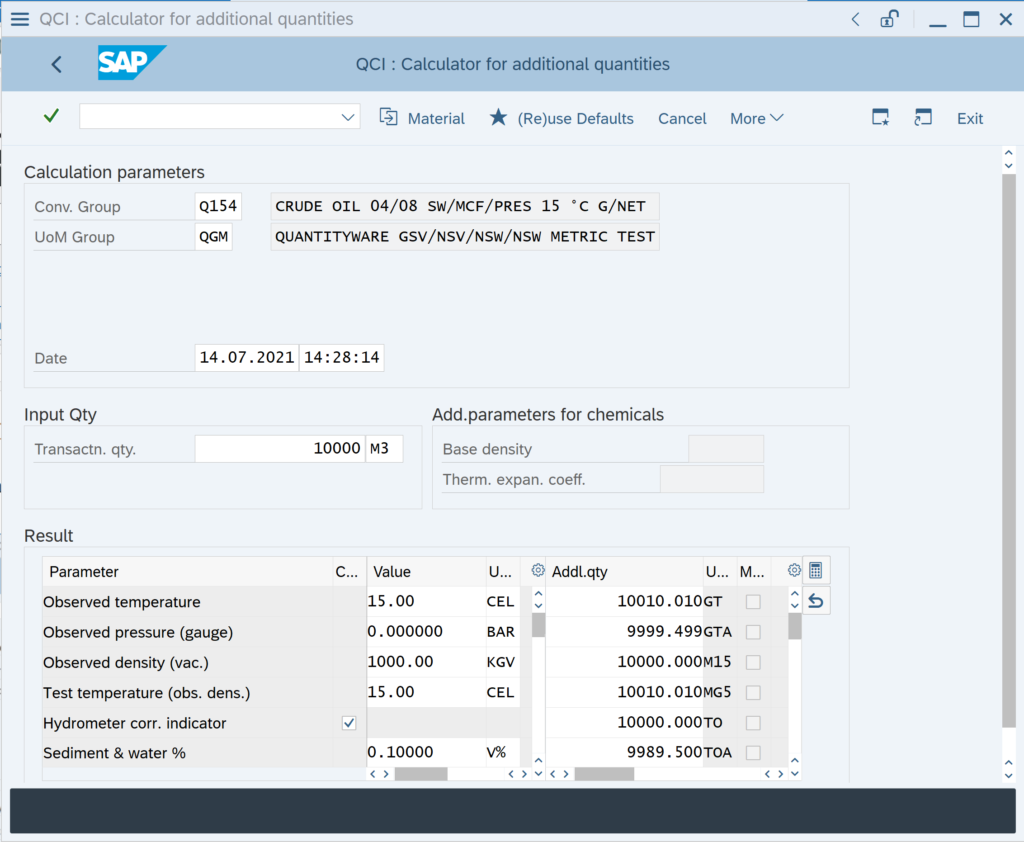Apparently, the gross ton value (UoM GT) is lager than expected, also the net ton (UoM TO) and the gross and net weights (UoM GTA and TOA) for a S&W fraction of 0.1 %. How can this be?

Answer: For S&W calculations, it is important to select the correct UoM for gross observed or gross standard volumes (GOV, GSV), which are typically the transaction quantities. By design, M3 is a NOV (Net Observed Volume) UoM. Thus, the calculation is performed “backwards”

### Our conversion group calculates the base density at 15 °C. We also need to report the base API gravity at 60 °F. Is this possible?

API MPMS Chapter 11.5 2009 provides the calculation logic for such calculations. It replaces the historical tables 3, 21 and 51 of ASTM D1250-80 Volume XI/XII.
This new logic has been implemented in an ABAP function for MQCI conversion groups, which requires a base temperature of either 15 °C or 60 °F. Template MQCI conversion group Q22A provides the configuration example: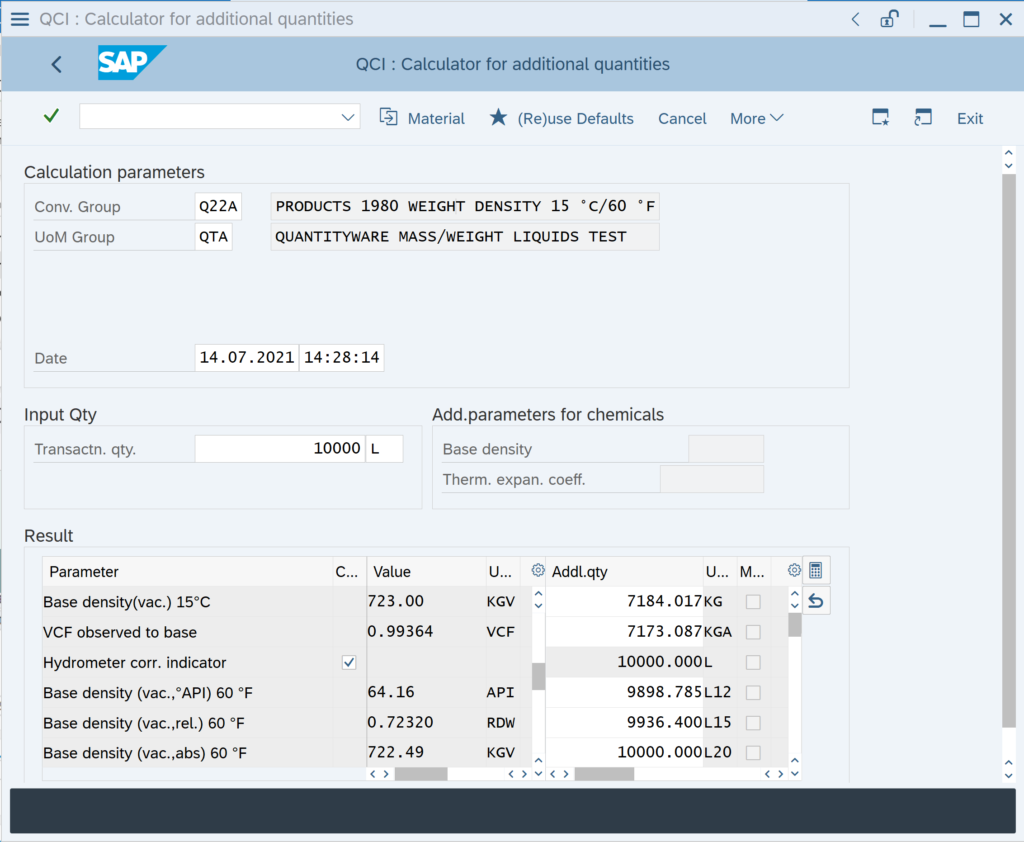MQCI ABAP function /QTYW/MQCI_CALC_TAB3_21_51_NEW needs to be assigned to the model function sequence: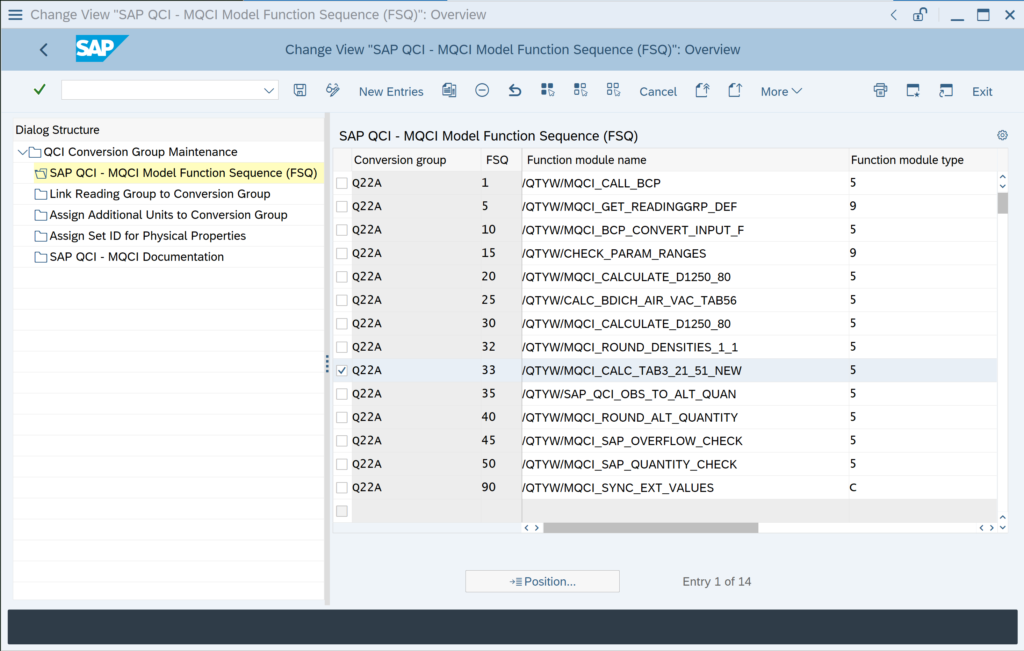### Can we enter the density for one product in different density types (°API, relative density, absolute density)?

Yes, all BCP MQCI conversion groups can be configured in such a manner.

Example: BCP Template Conversion Group Q210:

API Gravity: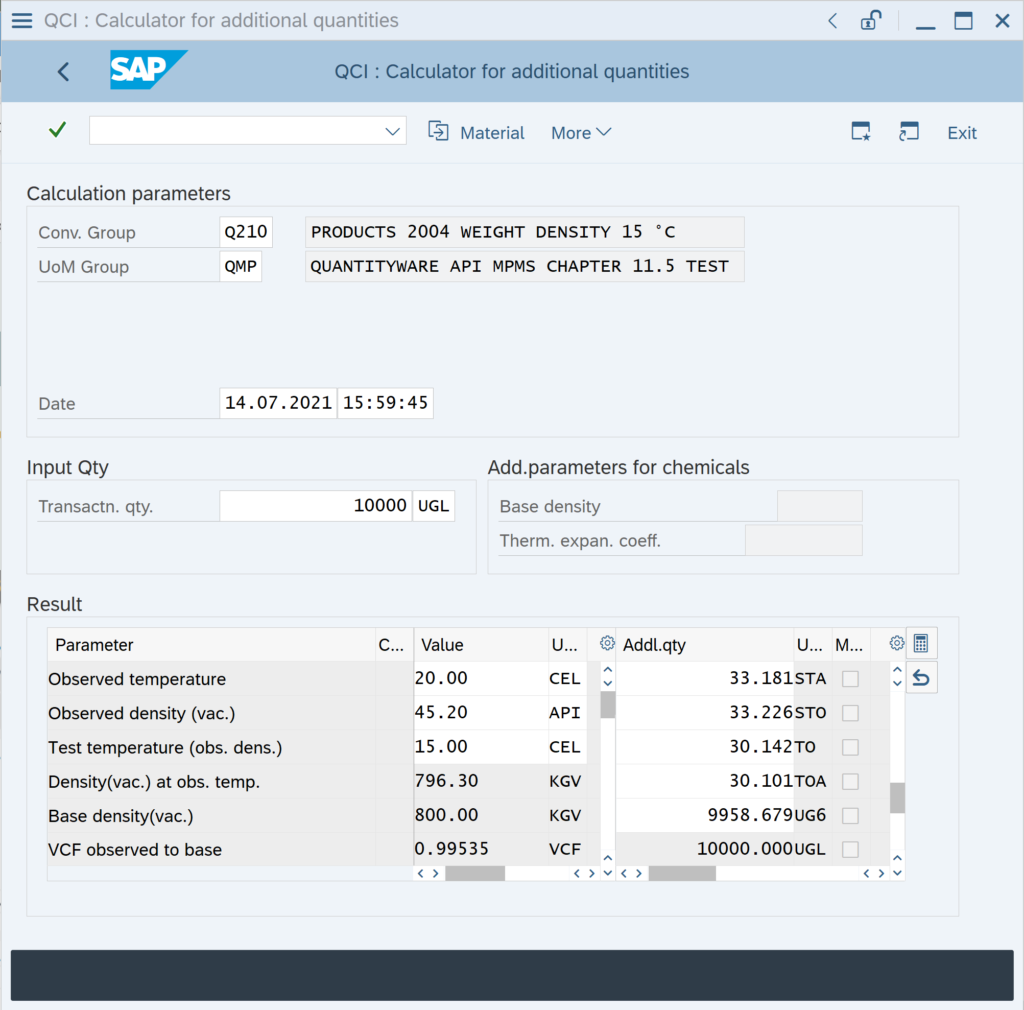Absolute Density: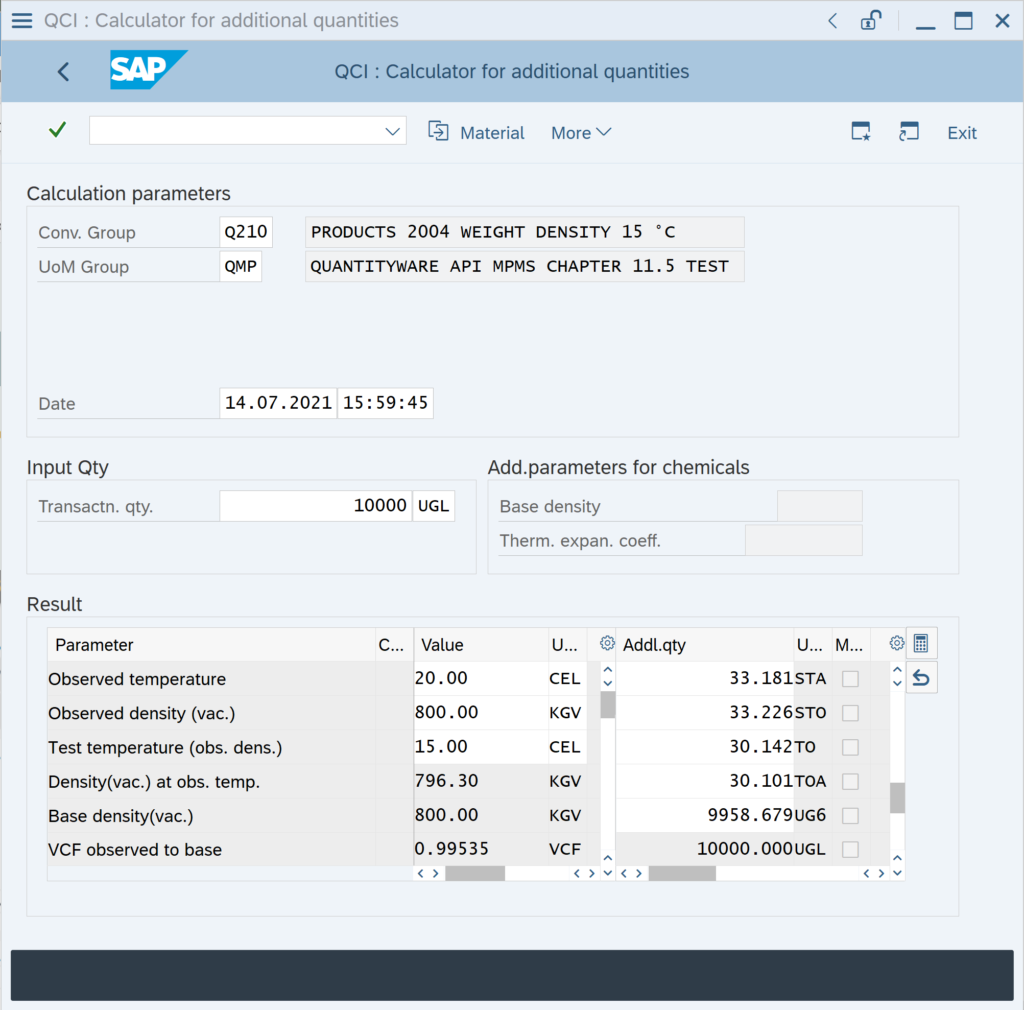Relative Density: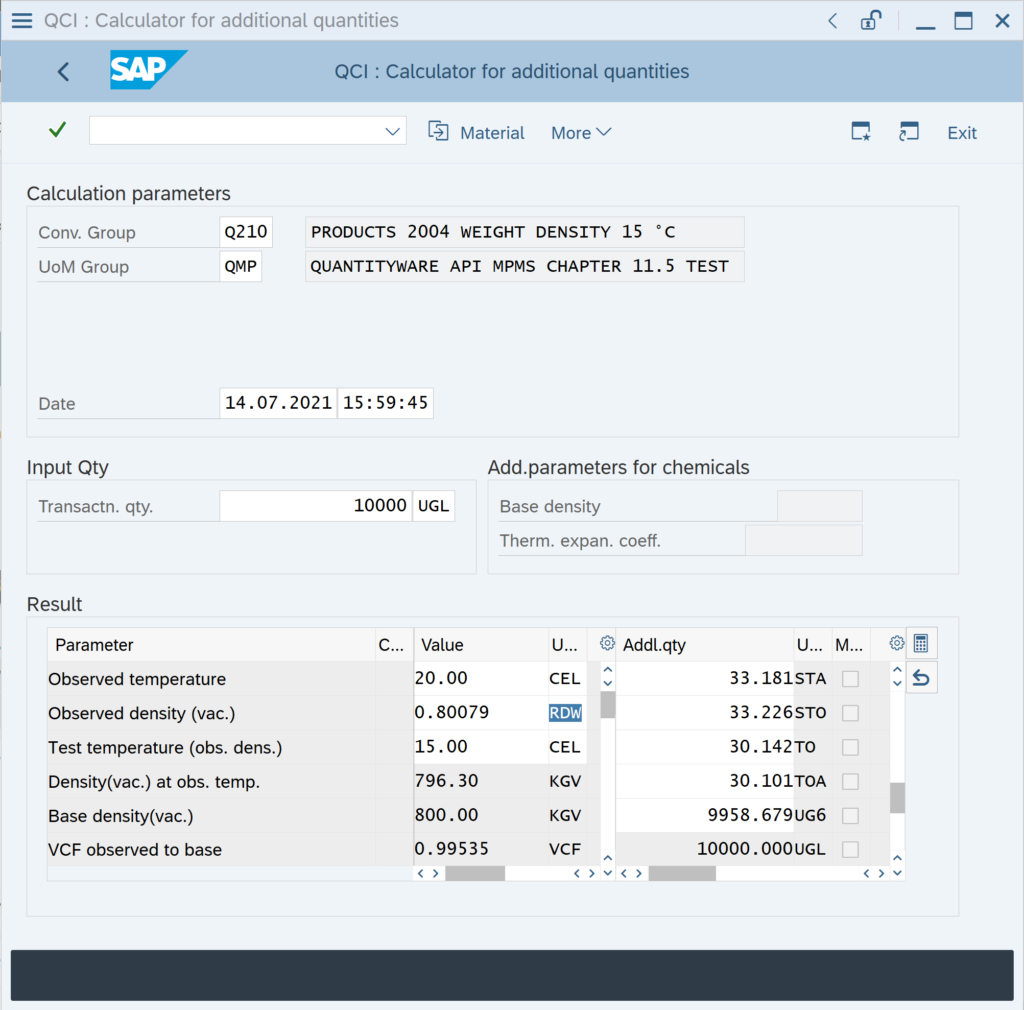In order to enable this flexible density type data entry, you simply add MQCI function module

/QTYW/MQCI_CON_TDICH_VAC_DNTYP with FMTYP 7

to the conversion group function module sequence: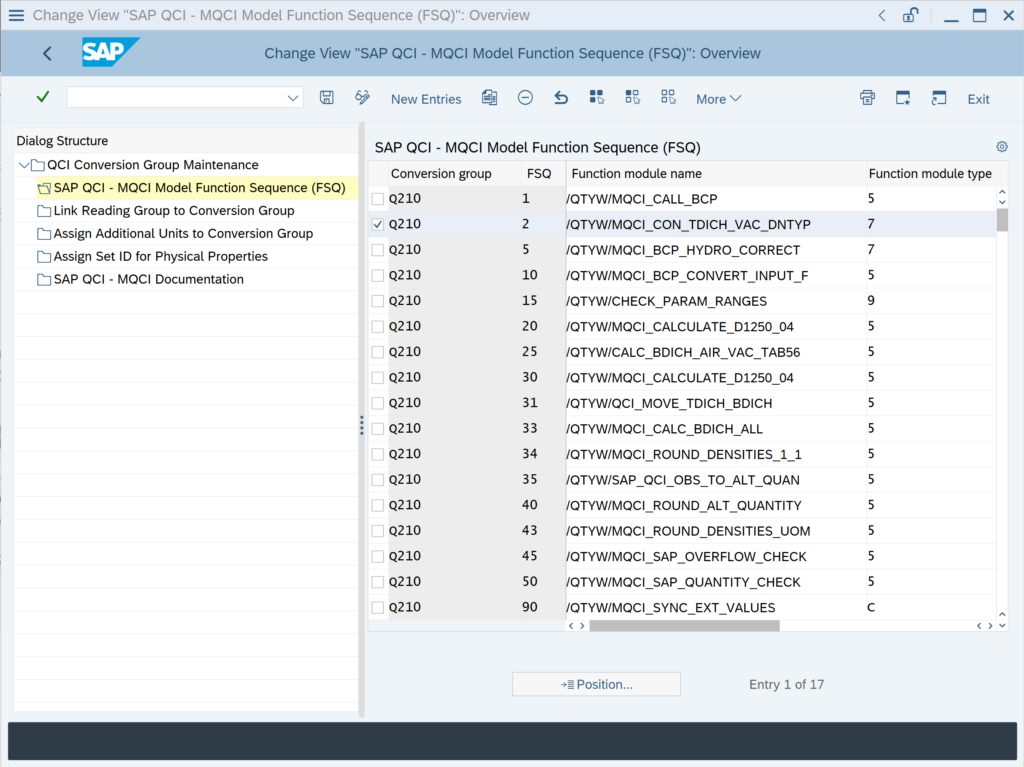### We need to report our product density in absolute density, relative density and API gravity. Is this possible?

Our customer requires reporting of calculated base density values in three density types; all at the conversion group base temperature. How can this be achieved?

All MQCI conversion groups support such calculations. BCP configuration template conversion group Q210 can be used as a reference: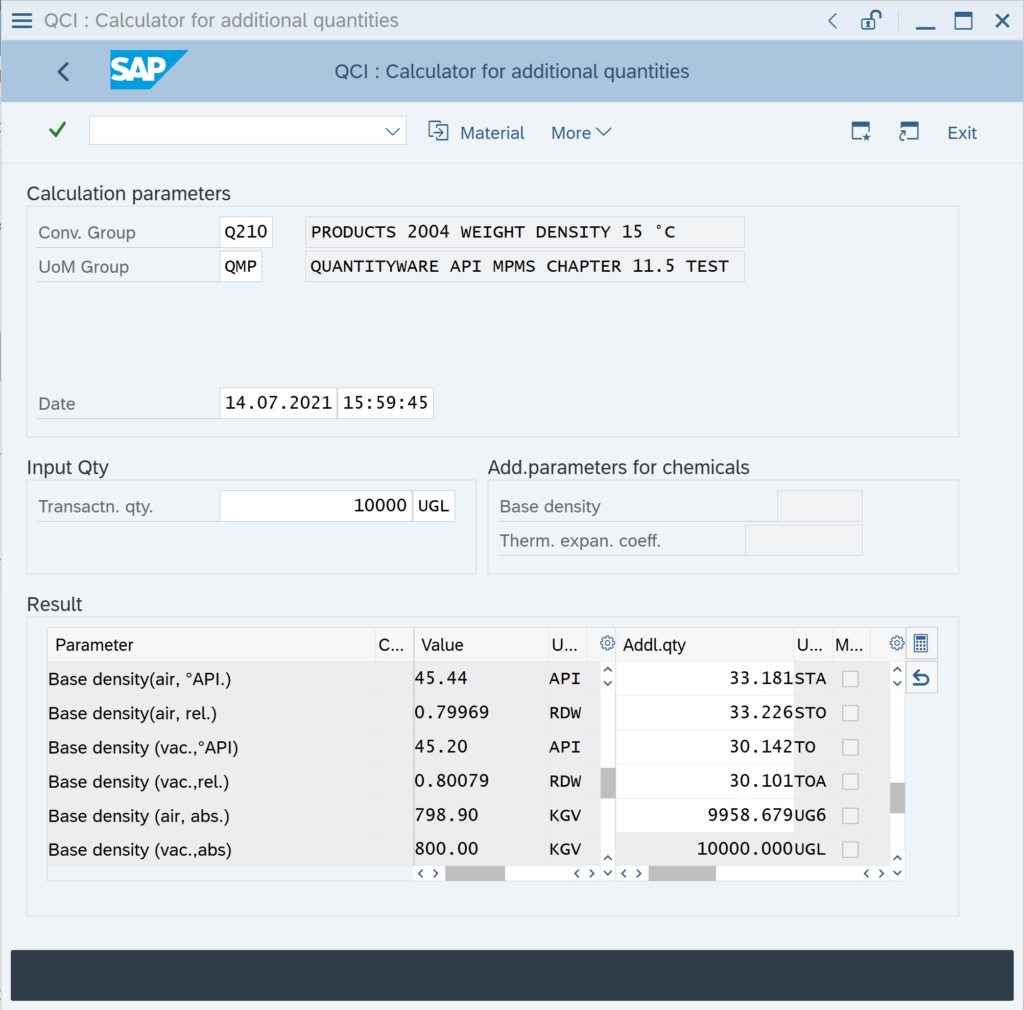To achieve this, you simply add the function

/QTYW/MQCI_CALC_BDICH_ALL

to the MQCI function module sequence: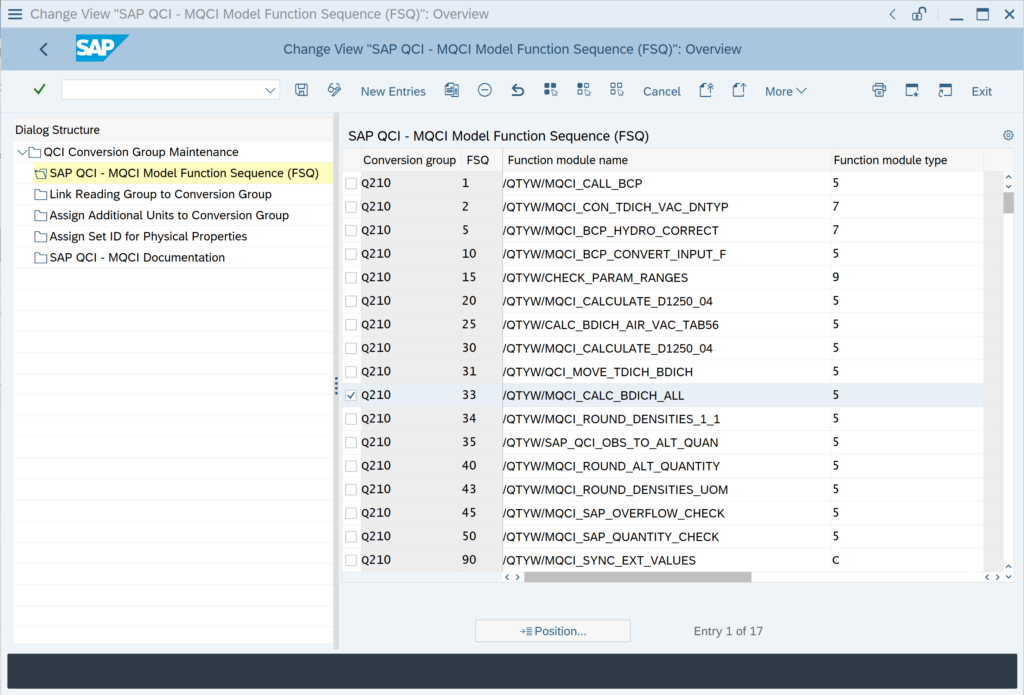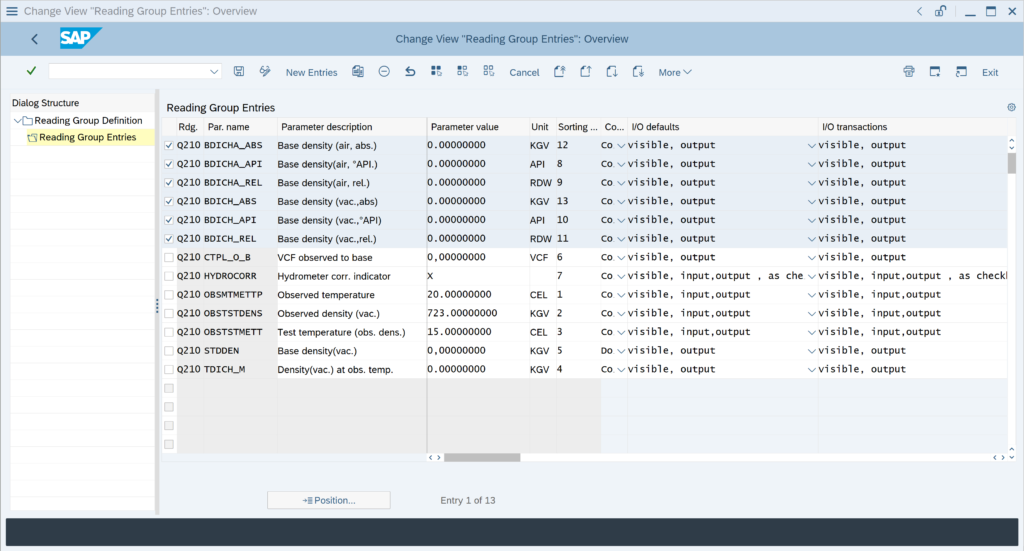Note: If you require calculation of e.g.

### We directly want to enter the base density of our products, w/o having to enter a test temperature. Is this possible?

Yes, all BCP MQCI conversion groups can be configured in such a manner.

Example: BCP Template Conversion Group Q2A1: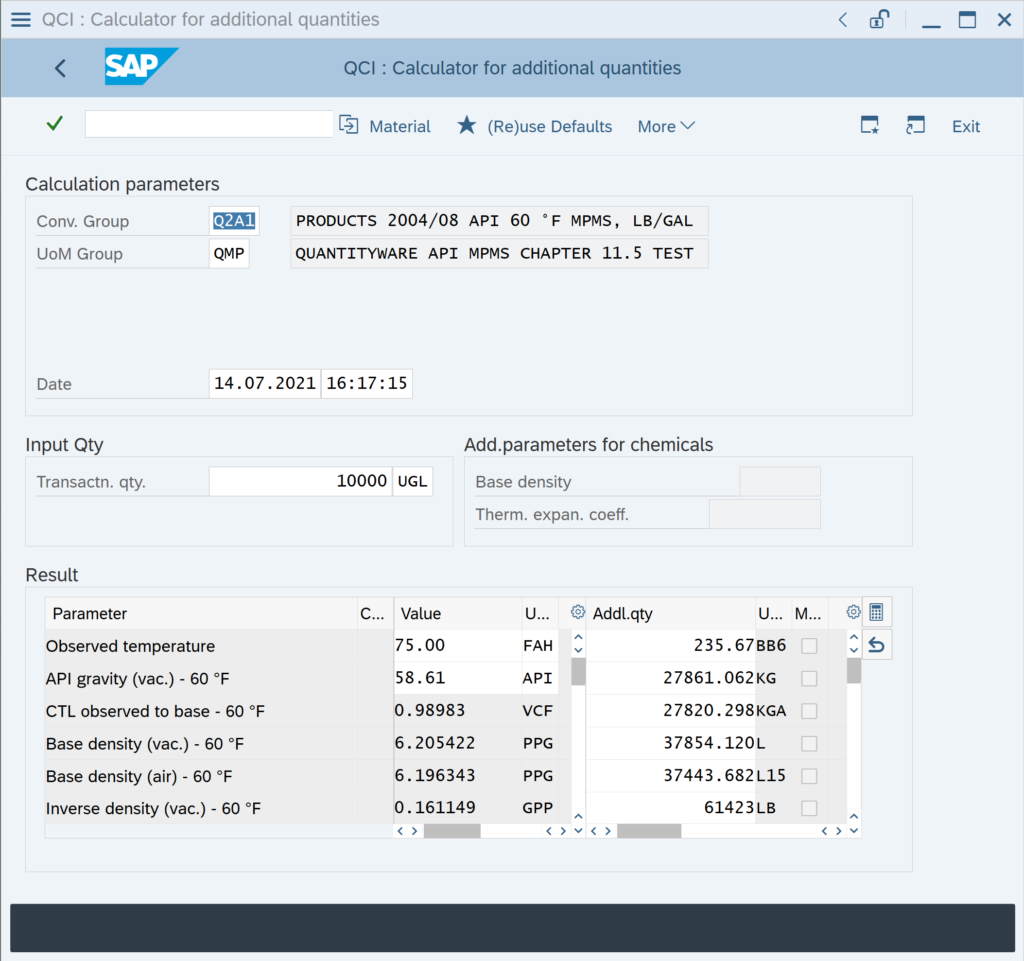Here you only enter the observed product temperature and the base density  as input parameters. Then, the VCF and all densities in air values are calculated, as well as all quantity values.

Technically, this is achieved by adding MQCI Function Module

/QTYW/MQCI_BCP_PROD_DEFAULT with FMTYP 9

to the Function Module Sequence (FSQ) in the configuration settings: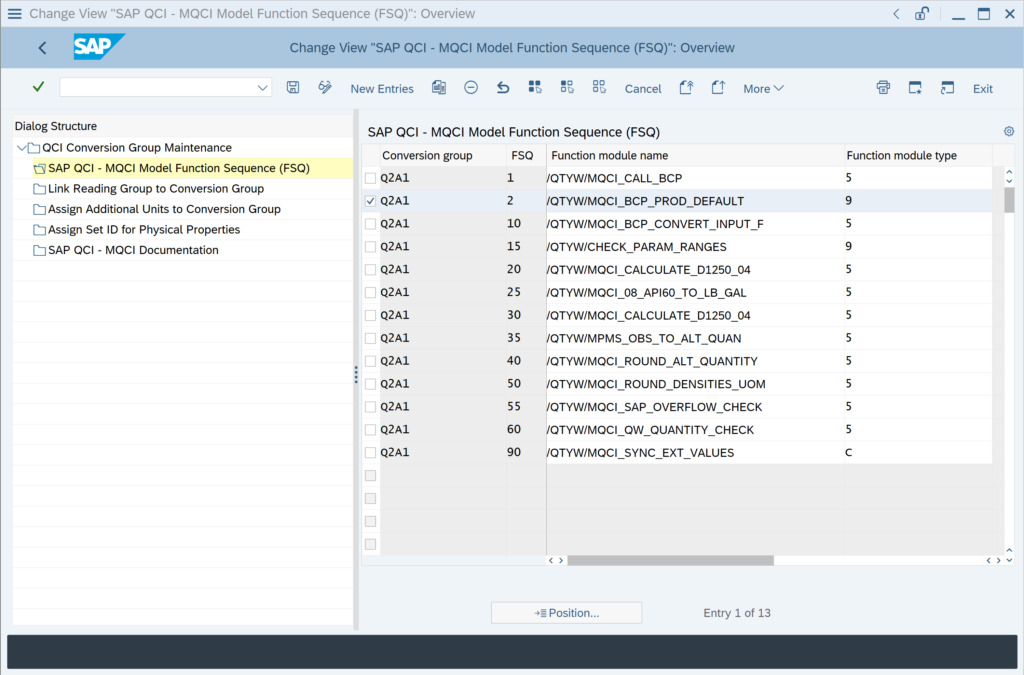Then you may remove the test temperature parameter from the reading group,

### How can I change the number of decimals (rounding) of my VCF / CTL ?

The Volume Correction Factor (VCF), also known as Correction for the effect of Temperature on Liquid (CTL), is defined in various measurement standards – whereby product specific standards and historical versions need to be considered. Therein, the calculation procedure and the number of decimals, to which the VCF shall be rounded, are specified.

Historically, volume correction factors have been defined as printed tables – starting with ASTM D1250-80, implementation procedures for software programs became the primary standard, not the printed tables any more.

Examples: Printed tables print the VCF with 3 or 4 decimals (e.g.

### We do not require the hydrometer correction. Can we remove it from our conversion group configuration?

The hydrometer correction may only be required, if you enter an observed density that is determined with a glass hydrometer. If you utilize an ASTM D1250-04 implementation, you may simply remove the hydrometer function (and also remove the hydrometer indicator from the reading group):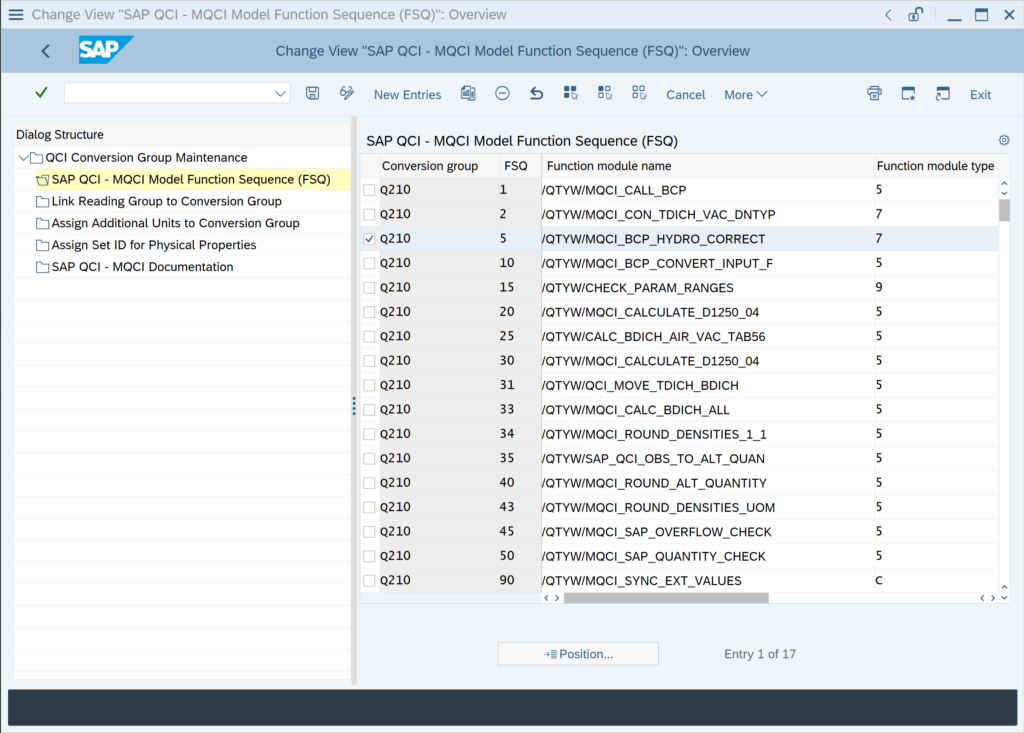ASTM D1250-80 implementations contain a built-in (by measurement standard definition) hydrometer correction logic, which requires that you actively turn the correction off if not required – read note 000026 for all important details.

### I have set my quantity value UoM L15 and KG to be rounded to zero decimals, but it is not working. What can I do?

The UoM real rounding settings are considered for all MQCI conversion groups (where rounding function

/QTYW/MQCI_ROUND_ALT_QUANTITY

is assigned). To define the real rounding for a UoM, go to the PMC and navigate to: More -> Goto -> UoM Rounding: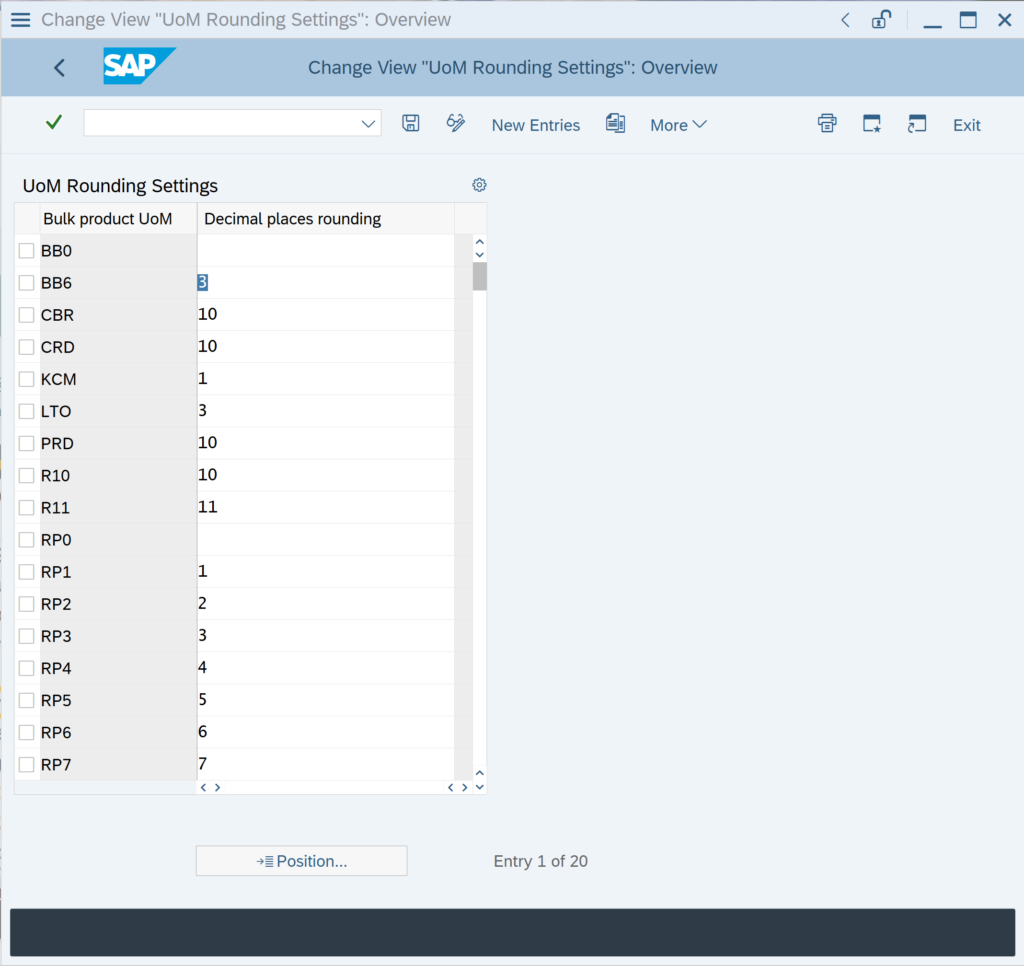and maintain the number of decimals for your UoM that shall be rounded accordingly.

NOTE: In the main UoM transaction CUNI, you define the number of display decimals for your UoM only. Thus, if you e.g. set the display UoM decimals in CUNI to 1,

### My rounding settings for the density values for an MQCI conversion group are not considered for the calculation. How can I fix this?

You have copied e.g. template conversion group Q1A1 to Z1A1. For the density UoM PPG (pounds per gallon) you have maintained a rounding to 4 decimals; business requires that that rounded value is utilized for the calculation of masses.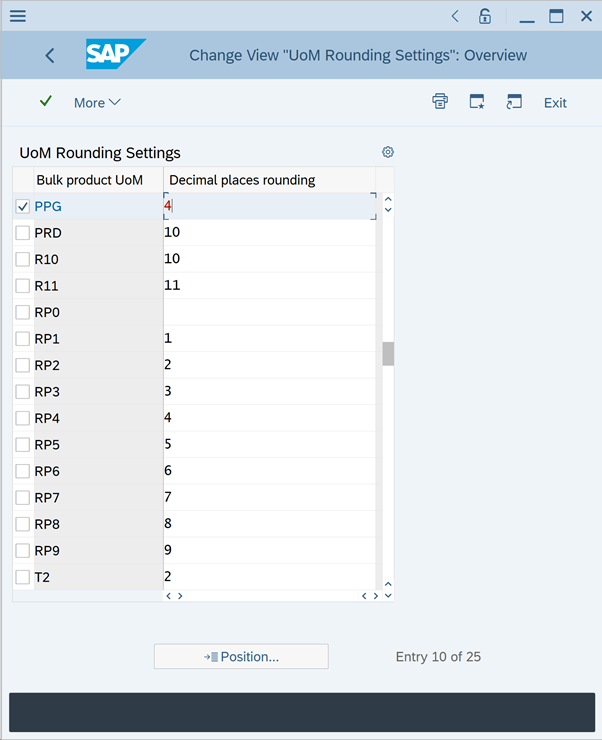A simple test calculation with Q1A1 shows the issue: The expected value for UoM LB is 620 540.00 LB; however, the value is calculated using the non-rounded density (while the rounded value is displayed):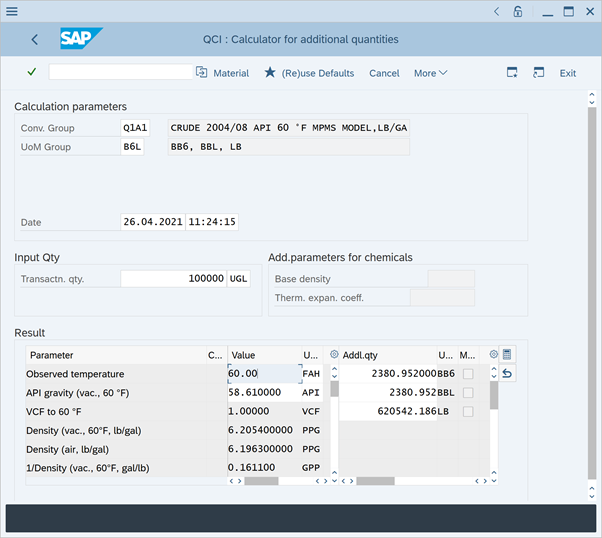This is due to the fact, that the density rounding function /QTYW/MQCI_ROUND_DENSITIES_UOM is processed after the quantity conversion model function /QTYW/MPMS_OBS_TO_ALT_QUAN: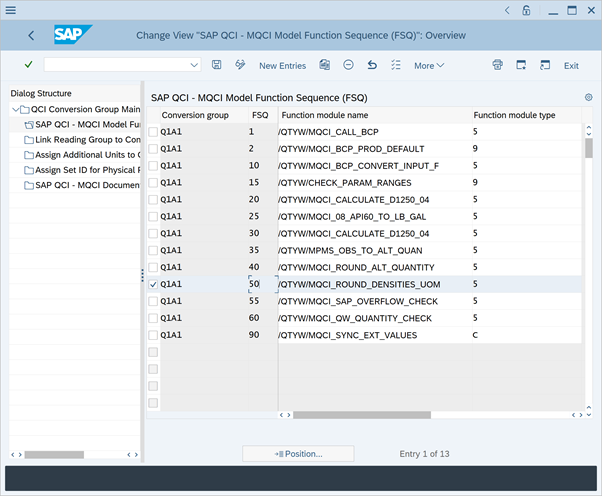If you position the rounding function before the quantity conversion model function: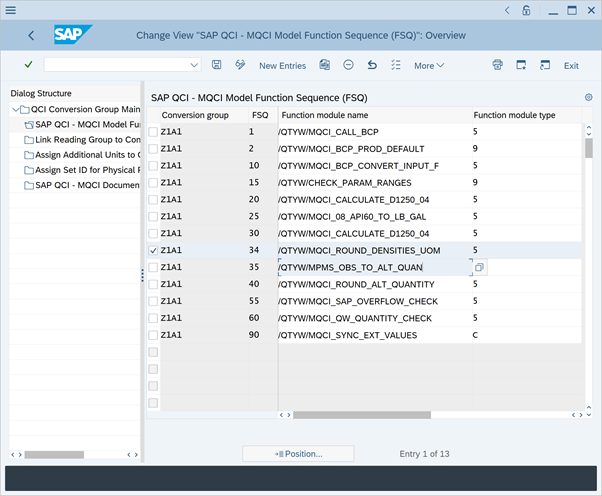the calculation is happening as expected: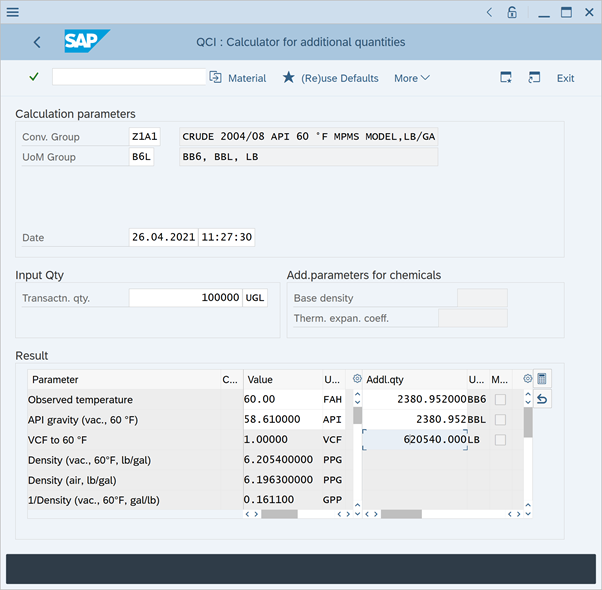The Q*** template conversion groups are configured to round the density values for reporting purposes,

### The system displays a volume correction factor (VCF) of 1 for any observed temperature. Why is that?

MQCI conversion groups can be configured to display the volume correction factor (VCF / CTL / CTPL) from observed temperature to base temperature. The observed temperature (also known as material temperature) is associated with the transaction quantity of the product.

If you enter a transaction quantity with a UoM that has a temperature value assigned (GSV or NSV), the VCF is always calculated taking that temperature as observed temperature. By design, you are allowed to enter such a transaction quantity UoM.

Example 1 – 100 000 L15 :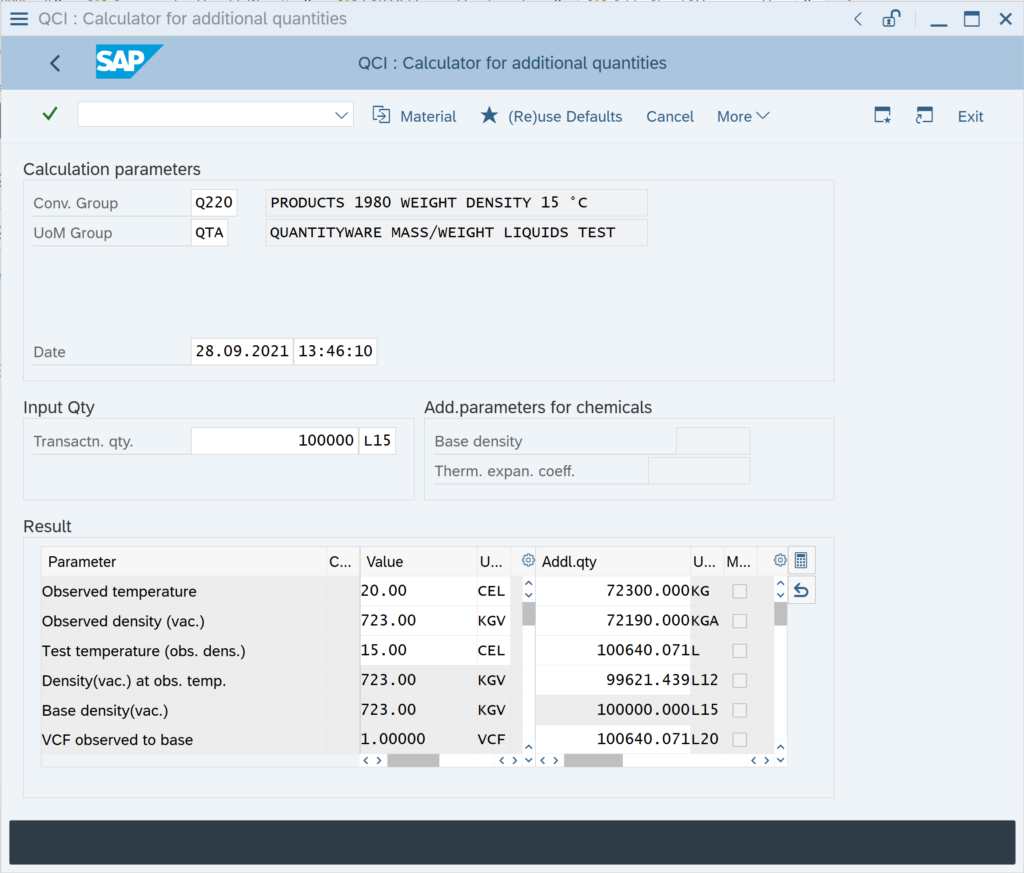The 15 °C assigned to L15 “overwrite”

### My customer requires ASTM Table 1 UoM conversion factors. What are the configuration steps?

For MQCI conversion groups, you simply activate the ASTM Table 1 conversion factors and ASTM Table 1 version in the conversion group detail settings: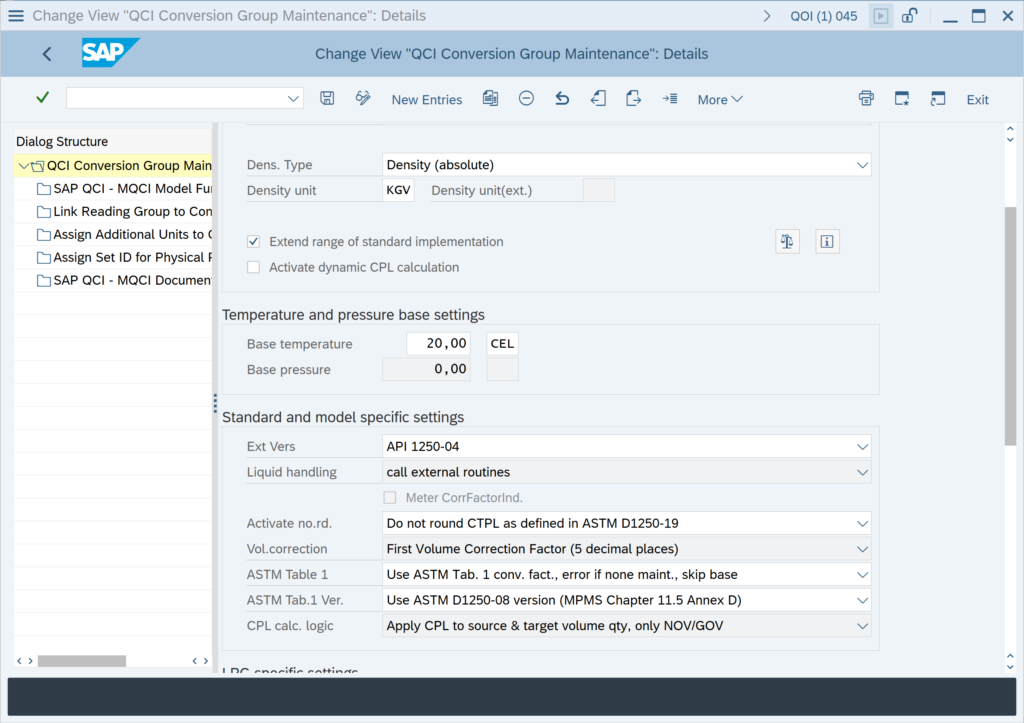With the QuantityWare BCS configuration templates, ASTM Table 1 conversion factors are delivered, which are accessible in your client 045 after activation of the relevant Business Configuration (BC) set. Read Note 000055 for all important additional configuration details.

### My client wants to use MQCI conversion groups, where kg, lb, to, sto UoM are calculated as weights - is this possible?

Yes. The BCS configuration template provides example MQCI conversion groups that are configured such that UoM of SAP dimension ID MASS are calculated as weights (which is also the case for SAP QCI conversion groups if the air buoyancy indicator is set):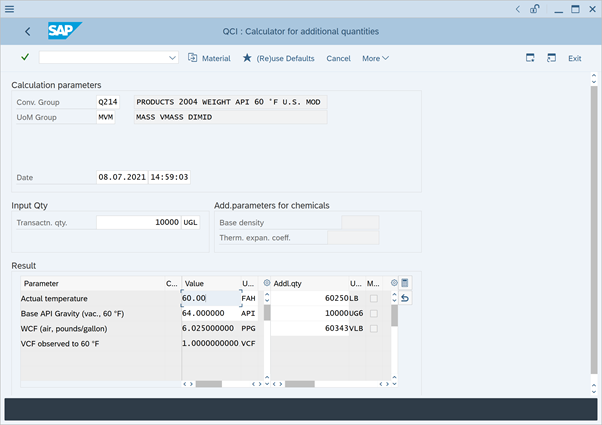The LB value is the weight of the 10 000 gallons of product. The configuration to achieve this requires 2 (plus 1) steps:

You assign the SAP Dimension IDs for weight (and mass) DIMID: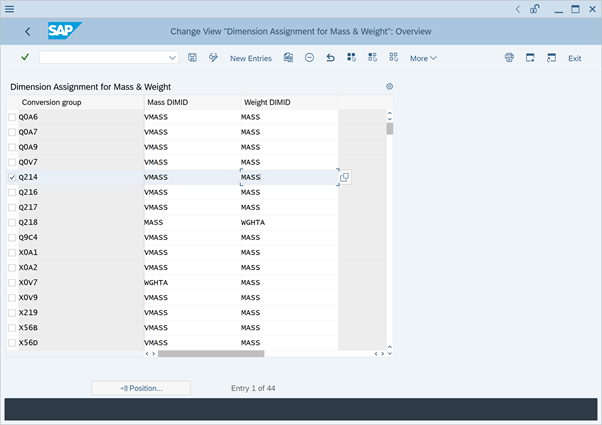You assign marker function /QTYW/MQCI_WEIGHT_MASS to the MQCI model function sequence: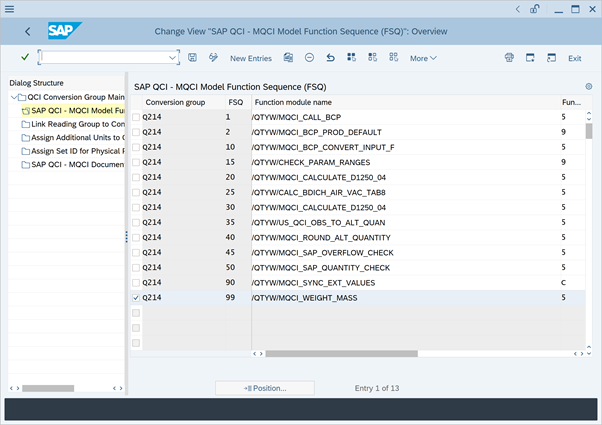Depending on the MQCI calculation model,

### Can I configure my products conversion groups, such that gross and net quantities are being calculated?

QuantityWare BCP contains four crude oil calculation models, that are utilized to distinguish between gross and net quantity values (e.g. gross standard or observed volume and net standard or observed volume volume).

These four models are assigned to crude oil BCP MQCI template conversion groups, e.g. Q154. For crude oil, the sediment & water fraction accounts to a difference of gross and net quantity values – see separate crude oil FAQ.

For products (e.g. gasoline, LPG, asphalt …), the gross and net quantity values are typically identical. Thus, the quantity conversion models assigned to such products are all net models and calculations for gross UoM are not supported.